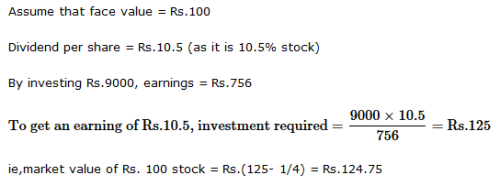Courses

# Test: Stocks And Shares- 1

## 10 Questions MCQ Test Quantitative Aptitude (Quant) | Test: Stocks And Shares- 1

Description
This mock test of Test: Stocks And Shares- 1 for CAT helps you for every CAT entrance exam. This contains 10 Multiple Choice Questions for CAT Test: Stocks And Shares- 1 (mcq) to study with solutions a complete question bank. The solved questions answers in this Test: Stocks And Shares- 1 quiz give you a good mix of easy questions and tough questions. CAT students definitely take this Test: Stocks And Shares- 1 exercise for a better result in the exam. You can find other Test: Stocks And Shares- 1 extra questions, long questions & short questions for CAT on EduRev as well by searching above.
QUESTION: 1

### Q. A man buys Rs. 20 shares paying 9% dividend. The man wants to have an interest of 12% on his money. What is the market value of each share?

Solution: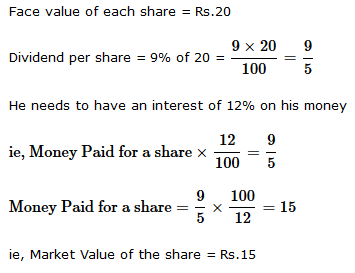QUESTION: 2

### A man invested Rs.1552 in a stock at 97 to obtain an income of Rs.128. What is the dividend from the stock?

Solution:

By investing Rs. 1552, income = Rs. 128.
By investing Rs. 97, income =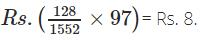Dividend = 8%

QUESTION: 3

### The cost price of a Rs. 100 stock at 4 discount, when brokerage is 1/5% is

Solution: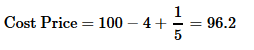QUESTION: 4

In order to obtain an income of Rs. 650 from 10% stock at Rs. 96, one must make an investment of

Solution: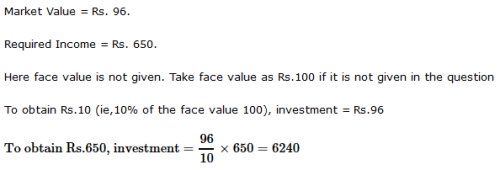QUESTION: 5

By investing in 50/3% stock at 64, one earns Rs. 1500. The investment made is

Solution: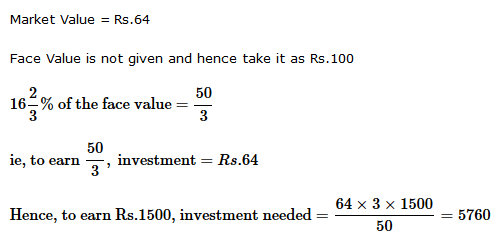QUESTION: 6

A man invested Rs. 4940 in Rs. 10 shares quoted at Rs. 9.50. If the rate of dividend be 14%, his annual income is

Solution:QUESTION: 7

A man invests some money partly in 12% stock at 105 and partly in 8% stock at 88. To obtain equal dividends from both, he must invest the money in the ratio:

Solution:

In case of stock1, if he invest Rs.105, he will get a dividend of Rs.12 (assume face value = 100)

In case of stock2, if he invest Rs.88, he will get a dividend of Rs.8 (assume face value = 100)

ie, if he invest Rs.(88*12)/8, he will get a dividend of Rs.12

Required ratio = 105 : (88 × 12)/8 = 105 : (11 × 12) = 35 : (11 × 4) = 35 : 44

QUESTION: 8

A man bought 40 shares of Rs. 60 at 5 discount, the rate of dividend being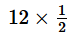%The rate of interest obtained is

Solution: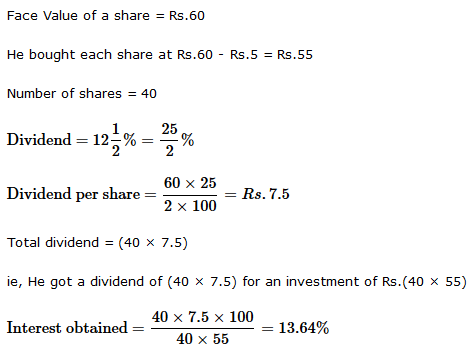QUESTION: 9

By investing Rs. 1800 in 9% stock, Syam earns Rs. 120. The stock is then quoted at

Solution: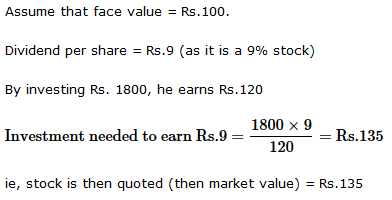QUESTION: 10

The market value of a 10.5% stock, in which an income of Rs. 756 is derived by investing Rs. 9000, brokerage being 14 %, is:

Solution: